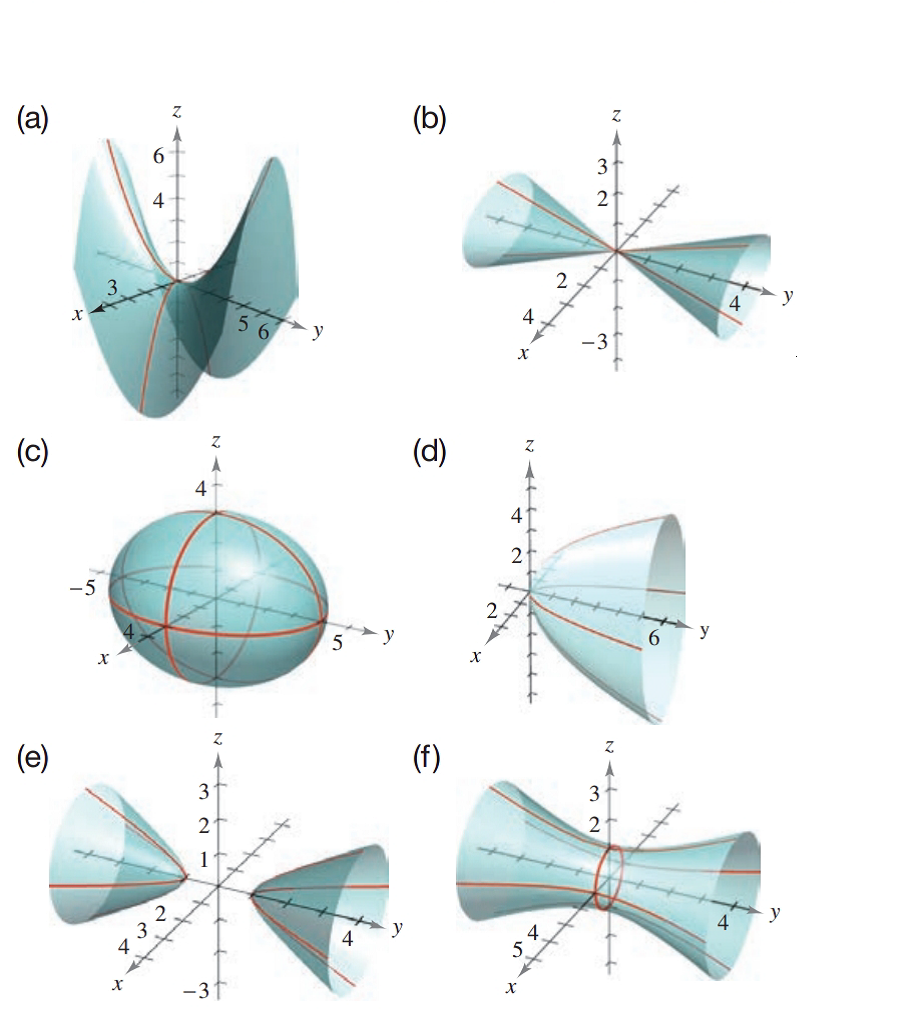Chapter 7.2, Problem 27E### Calculus: An Applied Approach (Min...

10th Edition
Ron Larson
ISBN: 9781305860919

#### Solutions

Chapter
Section### Calculus: An Applied Approach (Min...

10th Edition
Ron Larson
ISBN: 9781305860919
Textbook Problem
1 views

# Matching In Exercises 23-28, match the equation with its graph. [The graphs are labeled (a)-(f).]4 x 2 − 4 y 2 + z 2 = 0

To determine

The quadratic surface represented by the equation 4x24y2+z2=0 and its correct graph from the provided graph.

Explanation

Given Information:

The provided quadratic surface equation is 4x24y2+z2=0 and the provided option for matching as follows:

Consider the provided equation 4x24y2+z2=0

Rewrite the equation as in standard format,

4x29y+z2=04x2+z2=4y4x24+z24=4y4x21+z24=4y24

The above equation can be written as,

x2

### Still sussing out bartleby?

Check out a sample textbook solution.

See a sample solution

#### The Solution to Your Study Problems

Bartleby provides explanations to thousands of textbook problems written by our experts, many with advanced degrees!

Get Started

#### Convert the expressions in Exercises 6584 to power form. 35x2

Finite Mathematics and Applied Calculus (MindTap Course List)

#### In Exercises 73-80, find the indicated limits, if they exist. 79. limxx5x3+x1x6+2x2+1

Applied Calculus for the Managerial, Life, and Social Sciences: A Brief Approach

#### 28. Write the interval corresponding to .

Mathematical Applications for the Management, Life, and Social Sciences